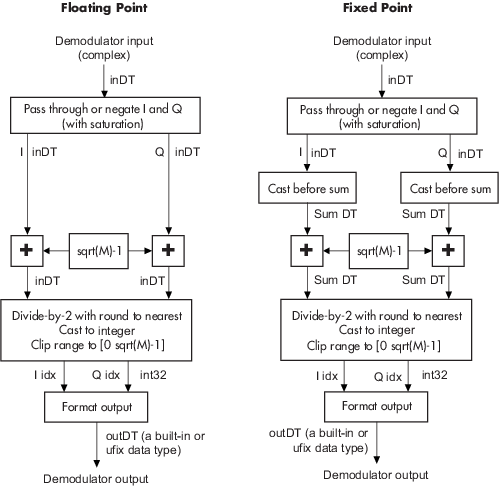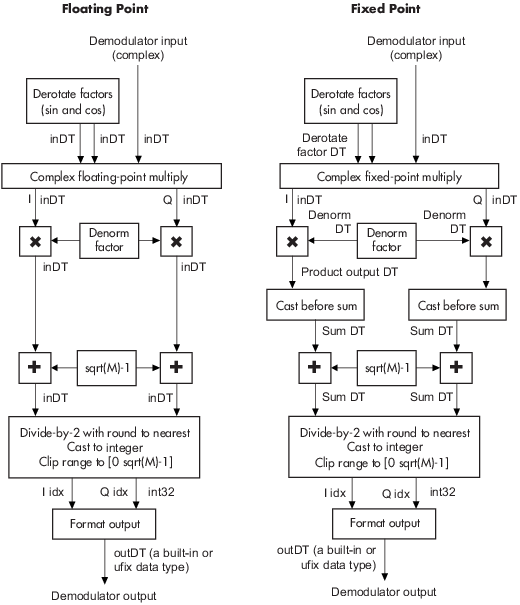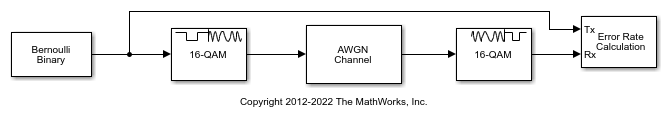Documentation

This is machine translation

Mouseover text to see original. Click the button below to return to the English version of the page.

Rectangular QAM Demodulator Baseband

Demodulate rectangular-QAM-modulated data

Library

AM, in Digital Baseband sublibrary of ModulationDescription

The Rectangular QAM Demodulator Baseband block demodulates a signal that was modulated using quadrature amplitude modulation with a constellation on a rectangular lattice.

Note

All values of power assume a nominal impedance of 1 ohm.

The signal constellation has M points, where M is the M-ary number parameter. M must have the form 2K for some positive integer K. The block scales the signal constellation based on how you set the Normalization method parameter. For details, see the reference page for the Rectangular QAM Modulator Baseband block.

This block accepts a scalar or column vector input signal. For information about the data types each block port supports, see the Supported Data Types table on this page.

Hard Decision Algorithm

The demodulator algorithm maps received input signal constellation values to M-ary integer I and Q symbol indices between 0 and $\sqrt{\text{M}}-1$ and then maps these demodulated symbol indices to formatted output values.

The integer symbol index computation is performed by first derotating and scaling the complex input signal constellation (possibly with noise) by a derotate factor and denormalization factor, respectively. These factors are derived from the Phase offset, Normalization method, and related parameters. These derotated and denormalized values are added to $\sqrt{\text{M}}-1$ to translate them into an approximate range between 0 and $2×\left(\sqrt{\text{M}}-1\right)$ (plus noise). The resulting values are then rescaled via a divide-by-two (or, equivalently, a right-shift by one bit for fixed-point operation) to obtain a range approximately between 0 and $\sqrt{\text{M}}-1$ (plus noise) for I and Q. The noisy index values are rounded to the nearest integer and clipped, via saturation, and mapped to integer symbol values in the range [0 M-1]. Finally, based on other block parameters, the integer index is mapped to a symbol value that is formatted and cast to the selected Output data type.

The following figures contains signal flow diagrams for floating-point and fixed-point algorithm operation. The floating-point diagrams apply when the input signal data type is `double` or `single`. The fixed-point diagrams apply when the input signal is a signed fixed-point data type. Note that the diagram is simplified when Phase offset is a multiple of π/2, and/or the derived denormalization factor is 1.

Signal-Flow Diagrams with Trivial Phase Offset and Denormalization Factor Equal to 1Signal-Flow Diagrams with Nontrivial Phase Offset and Nonunity Denormalization FactorParameters

M-ary number

The number of points in the signal constellation. It must have the form 2K for some positive integer K.

Normalization method

Determines how the block scales the signal constellation. Choices are `Min. distance between symbols`, `Average Power`, and ```Peak Power```.

Minimum distance

This parameter appears when Normalization method is set to `Min. distance between symbols`.

The distance between two nearest constellation points.

Average power, referenced to 1 ohm (watts)

The average power of the symbols in the constellation, referenced to 1 ohm. This field appears only when Normalization method is set to `Average Power`.

Peak power, referenced to 1 ohm (watts)

The maximum power of the symbols in the constellation, referenced to 1 ohm. This field appears only when Normalization method is set to `Peak Power`.

The rotation of the signal constellation, in radians.

Constellation ordering

Determines how the block assigns binary words to points of the signal constellation. More details are on the reference page for the Rectangular QAM Modulator Baseband block.

Selecting `User-defined` displays the field Constellation mapping, allowing for user-specified mapping.

Constellation mapping

This parameter appears when `User-defined` is selected in the pull-down list Constellation ordering.

This is a row or column vector of size M and must have unique integer values in the range [0, M-1]. The values must be of data type `double`.

The first element of this vector corresponds to the top-leftmost point of the constellation, with subsequent elements running down column-wise, from left to right. The last element corresponds to the bottom-rightmost point.

Output type

Determines whether the block produces integers or binary representations of integers.

If set to `Integer`, the block produces integers.

If set to `Bit`, the block produces a group of K bits, called a binary word, for each symbol, when Decision type is set to ```Hard decision```. If Decision type is set to `Log-likelihood ratio` or ```Approximate log-likelihood ratio```, the block outputs bitwise LLR and approximate LLR, respectively.

Decision type

This parameter appears when `Bit` is selected in the pull-down list Output type.

Specifies the use of hard decision, LLR, or approximate LLR during demodulation. See Exact LLR Algorithm and Approximate LLR Algorithm in the Communications Toolbox™ User's Guide for algorithm details.

Noise variance source

This parameter appears when ```Approximate log-likelihood ratio``` or `Log-likelihood ratio` is selected for Decision type.

When set to `Dialog`, the noise variance can be specified in the Noise variance field. When set to `Port`, a port appears on the block through which the noise variance can be input.

Noise variance

This parameter appears when the Noise variance source is set to `Dialog` and specifies the noise variance in the input signal. This parameter is tunable in normal mode, Accelerator mode and Rapid Accelerator mode.

If you use the Simulink® Coder™ rapid simulation (RSIM) target to build an RSIM executable, then you can tune the parameter without recompiling the model. This is useful for Monte Carlo simulations in which you run the simulation multiple times (perhaps on multiple computers) with different amounts of noise.

The LLR algorithm involves computing exponentials of very large or very small numbers using finite precision arithmetic and would yield:

• `Inf` to `-Inf` if Noise variance is very high

• `NaN` if Noise variance and signal power are both very small

In such cases, use approximate LLR, as its algorithm does not involve computing exponentials.Output

When the parameter is set to ```'Inherit via internal rule'``` (default setting), the block will inherit the output data type from the input port. The output data type will be the same as the input data type if the input is of type `single` or `double`. Otherwise, the output data type will be as if this parameter is set to ```'Smallest unsigned integer'```.

When the parameter is set to ```'Smallest unsigned integer'```, the output data type is selected based on the settings used in the Hardware Implementation pane of the Configuration Parameters dialog box of the model. If `ASIC/FPGA` is selected in the Hardware Implementation pane, the output data type is the ideal minimum size, i.e., `ufix(1)` for bit outputs, and `ufix(ceil(log2(M)))` for integer outputs. For all other selections, it is an unsigned integer with the smallest available word length large enough to fit the ideal minimum size, usually corresponding to the size of a char (e.g., `uint8`).

For integer outputs, this parameter can be set to ```Smallest unsigned integer```, `int8`, `uint8`, `int16`, `uint16`, `int32`, `uint32`, `single`, and `double`. For bit outputs, the options are `Smallest unsigned integer`, `int8`, `uint8`, `int16`, `uint16`, `int32`, `uint32`, `boolean`, `single`, or `double`.

Derotate factor

This parameter only applies when the input is fixed-point and Phase offset is not a multiple of π/2.

This can be set to `Same word length as input` or `Specify word length`, in which case a field is enabled for user input.

Denormalization factor

This parameter only applies when the input is fixed-point and the derived denormalization factor is nonunity (not equal to 1). This scaling factor is derived from Normalization method and other parameter values in the block dialog.

This can be set to `Same word length as input` or `Specify word length`, in which case a field is enabled for user input. A best-precision fraction length is always used.

Product output

This parameter only applies when the input is a fixed-point signal and there is a nonunity (not equal to 1) denormalized factor. It can be set to `Inherit via internal rule` or ```Specify word length```, which enables a field for user input.

Setting to `Inherit via internal rule` computes the full-precision product word length and fraction length. Internal Rule for Product Data Types (DSP System Toolbox) in DSP System Toolbox™ User's Guide describes the full-precision Product output internal rule.

Setting to `Specify word length` allows you to define the word length. The block computes a best-precision fraction length based on the word length specified and the pre-computed worst-case (min/max) real world value Product output result. The worst-case Product output result is precomputed by multiplying the denormalized factor with the worst-case (min/max) input signal range, purely based on the input signal data type.

The block uses the Rounding mode when the result of a fixed-point calculation does not map exactly to a number representable by the data type and scaling storing the result. For more information, see Rounding Modes (DSP System Toolbox) or Rounding Mode: Simplest (Fixed-Point Designer).

Sum

This parameter only applies when the input is a fixed-point signal. It can be set to `Inherit via internal rule`, ```Same as product output```, or `Specify word length`, in which case a field is enabled for user input

Setting to `Inherit via internal rule` computes the full-precision sum word length and fraction length, based on the two inputs to the Sum in the fixed-point Hard Decision Algorithm signal flow diagram. The rule is the same as the fixed-point inherit rule of the internal Accumulator data type parameter in the Simulink Sum block.

Setting to `Specify word length` allows you to define the word length. A best precision fraction length is computed based on the word length specified in the pre-computed maximum range necessary for the demodulated algorithm to produce accurate results. The signed fixed-point data type that has the best precision fully contains the values in the range $2*\left(\sqrt{M}-1\right)$ for the specified word length.

Setting to `Same as product output` allows the Sum data type to be the same as the Product output data type (when Product output is used). If the Product output is not used, then this setting will be ignored and the `Inherit via internal rule` Sum setting will be used.

Examples

expand all

Modulate and demodulate a noisy QAM signal.

Open the QAM demodulation model.Run the simulation. The results are saved to the base workspace, where the variable `ErrorVec` is a 1-by-3 row vector. The BER is found in the first element.

Display the error statistics. For the Eb/No provided, 2 dB, the resultant BER is approximately 0.1. Your results may vary slightly.

```ans = 0.0948 ```

Increase the Eb/No to 4 dB. Rerun the simulation, and observe that the BER has decreased.

```ans = 0.0167 ```

Supported Data Types

PortSupported Data Types

Input

• Double-precision floating point

• Single-precision floating point

• Signed fixed–point when M-ary number is an even power of `2` and:

• Output type is `Integer`

• Output type is `Bit` and Decision type is `Hard-decision`

Var

• Double-precision floating point

• Single-precision floating point

Output

• Double-precision floating point

• Single-precision floating point

• Boolean when Output type is `Bit`

• 8-, 16-, and 32-bit signed integers

• 8-, 16-, and 32-bit unsigned integers

• ufix(1) in ASIC/FPGA when Output type is `Bit`

• $ufix\left({\mathrm{log}}_{2}M\right)$ in ASIC/FPGA when Output type is `Integer`

References

 Smith, Joel G., “Odd-Bit Quadrature Amplitude-Shift Keying,” IEEE Transactions on Communications, Vol. COM-23, March 1975, 385–389.